## Sunday, July 9, 2023

### (R) Bedford's Law

In today’s article, we will be discussing Benford’s Law, specifically as it is utilized as an applied methodology to assess financial documents for potential fraud:

First, a bit about the phenomenon which Benford sought to describe:

The discovery of Benford's law goes back to 1881, when the Canadian-American astronomer Simon Newcomb noticed that in logarithm tables the earlier pages (that started with 1) were much more worn than the other pages. Newcomb's published result is the first known instance of this observation and includes a distribution on the second digit, as well. Newcomb proposed a law that the probability of a single number N being the first digit of a number was equal to log(N + 1) − log(N).

The phenomenon was again noted in 1938 by the physicist Frank Benford, who tested it on data from 20 different domains and was credited for it. His data set included the surface areas of 335 rivers, the sizes of 3259 US populations, 104 physical constants, 1800 molecular weights, 5000 entries from a mathematical handbook, 308 numbers contained in an issue of Reader's Digest, the street addresses of the first 342 persons listed in American Men of Science and 418 death rates. The total number of observations used in the paper was 20,229. This discovery was later named after Benford (making it an example of Stigler's law).

Source: https://en.wikipedia.org/wiki/Benford%27s_law

So what does this actually mean in laymen’s terms?

Essentially, given a series of numerical elements from a similar source, we should expect certain leading digits to occur, and correspond to, a particular distribution patter.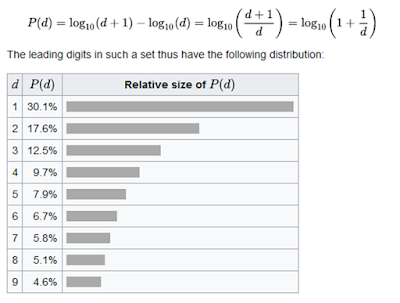If a series of elements perfectly corresponds with Benford’s Law, then the elements within the series should follow the above pattern as it pertains to leading digit frequency. Ex. Numbers which begin the number “1”, should occur 30.1% of the time. Numbers which begin with the number “2”, should occur 17.6% of the time. Numbers which begin with the number “3”, should occur 12.5% of the time.

The distribution is derived as follows: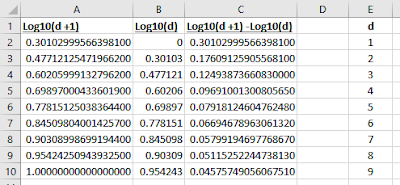The utilization of Benford’s Law is applicable to numerous scenarios:

1. Accounting fraud detection

2. Use in criminal trials

3. Election data

4. Macroeconomic data

5. Election data

6. Price digit analysis

7. Genome data

8. Scientific fraud detection

As it relates to screening for financial fraud, if the application of methodology related to the Benford’s Law Distribution returns a result in which the sample elements do not correspond with the distribution, then fraud is not necessarily the conclusion which we would immediately assume. However, the findings may indicate that additional data scrutinization is necessary.

Example:

Let’s utilize Benford’s Law to analyze Cloudflare’s (NET) Balance Sheet (12/31/2021).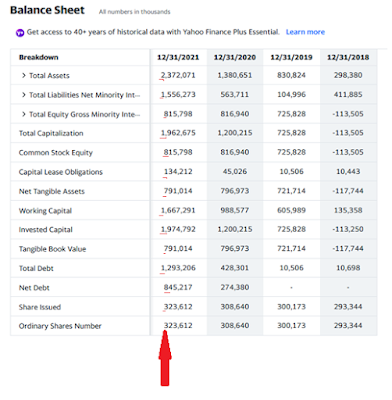Even though it’s an un-necessary step as it relates to our analysis, let’s first discern the frequency of each leading digit. These digits are underlined in red within the graphic above.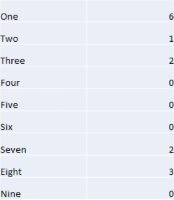What Benford’s Law will seeks to assess, is the comparison of leading digits as they occurred within our experiment, to our expectations as they exist within the Benford’s Law Distribution.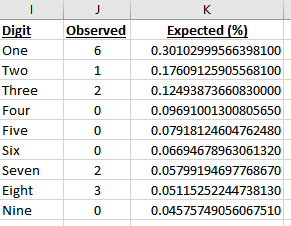The above table illustrates the frequency of occurrence of each leading digit within our analysis, versus the expected percentage frequency as stated by Benford’s Law.

Now let’s perform the analysis:

# H0: The first digits within the population counts follow Benford's law #

# H1: The first digits within the population counts do not follow Benford's law #

# requires benford.analysis #

library(benford.analysis)

# Element entries were gathered from Cloudflare’s (NET) Balance Sheet (12/31/2021) #

NET <- c(2372071.00, 1556273.00, 815798.00, 1962675.00, 815798.00, 134212.00, 791014.00, 1667291.00, 1974792.00, 791014.00, 1293206.00, 845217.00, 323612.00, 323612.00)

# Perform Analysis #

trends <- benford(NET, number.of.digits = 1, sign = "positive", discrete=TRUE, round=1)

# Display Analytical Output #

trends

# Plot Analytical Findings #

plot(trends)

Which provides the output:

Benford object:

Data: NET
Number of observations used = 14
Number of obs. for second order = 10
First digits analysed = 1

Mantissa:

Statistic Value
Mean 0.51
Var 0.11
Ex.Kurtosis -1.61
Skewness 0.25

The 5 largest deviations:

digits absolute.diff
1 8 2.28
2 1 1.79
3 2 1.47
4 4 1.36
5 7 1.19

Stats:

Pearson's Chi-squared test

data: NET
X-squared = 14.729, df = 8, p-value = 0.06464

Mantissa Arc Test

data: NET
L2 = 0.092944, df = 2, p-value = 0.2722

MAD Conformity - Nigrini (2012): Nonconformity
Distortion Factor: 8.241894

Remember: Real data will never conform perfectly to Benford's Law. You should not focus on p-values!

~ Graphical Output Provided by Function ~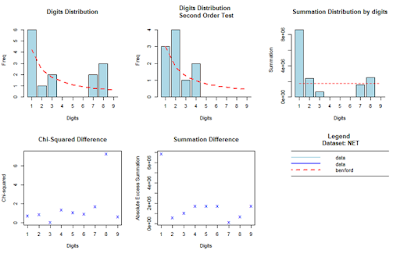(The most important aspects of the output are bolded)

Findings:

Pearson's Chi-squared test

data: NET
X-squared = 14.729, df = 8, p-value = 0.06464
Remember: Real data will never conform perfectly to Benford's Law. You should not focus on p-values!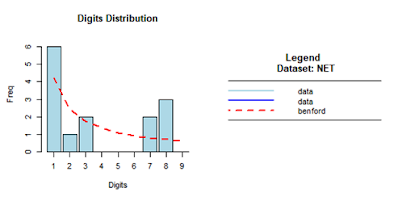A chi-square goodness of fit test was performed to examine whether the first digit of balance sheet items from the company Cloudflare (12/31/2021), adhere to Benford's law. Entries were found to be in adherence, with non-significance at the p < .05 level, χ2 (8, N = 14) = 14.73, p = 0.07.

As it relates to the graphic, in ideal circumstances, each blue data bar should have its uppermost portion touching the broken red line.

Example(2):

If you’d prefer to instead run the analysis simply as a chi-squared test which does not require the “benford.analysis” package, you can effectively utilize the following code. The image below demonstrates the concept being employed.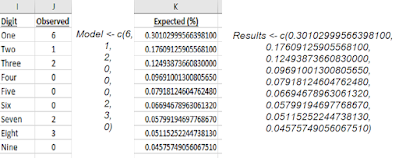Model <- c(6, 1, 2, 0, 0, 0, 2, 3, 0)

Results <- c(0.30102999566398100, 0.17609125905568100, 0.12493873660830000, 0.09691001300805650, 0.07918124604762480, 0.06694678963061320, 0.05799194697768670, 0.05115252244738130, 0.04575749056067510)

chisq.test(Model, p=Results, rescale.p = FALSE)

Which provides the output:

Chi-squared test for given probabilities

data: Model
X-squared = 14.729, df = 8, p-value = 0.06464

Which are the same findings that we encountered while performing the analysis previously.

That’s all for now! Stay studious, Data Heads!

-RD